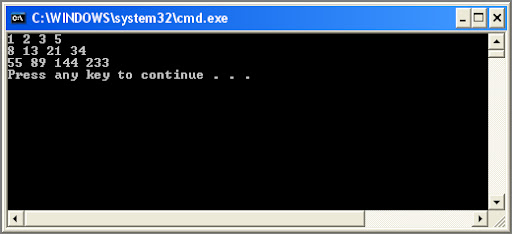## Saturday, February 16, 2013

### Basic Matrix Operations with VB.NET Part 1: Intro to Matrices and Arrays

In math a matrix is simply a table of values, generally drawn like this.Note a couple things here. The variable the matrix is assigned to is usually bold, and the table of values is usually between a set of brackets. The example matrix above is a 3 by 4 matrix, with three rows and four columns.

In VB.NET you can represent a matrix with a multidimensional array. The only real trick is to remember that you start counting rows and columns with 1 in a matrix, and you start counting rows and columns with zero in an array. So a 3 by 4 matrix is a 2 by 3 array. It will have the same number of values in it. The matrix has 1,2,3 rows and 1,2,3,4 columns; the array has 0,1,2 rows and 0,1,2,3 columns. Here is the code for the above example matrix.

Sub Main()
Dim A(2, 3) As Integer
A(0, 0) = 1
A(0, 1) = 2
A(0, 2) = 3
A(0, 3) = 5
A(1, 0) = 8
A(1, 1) = 13
A(1, 2) = 21
A(1, 3) = 34
A(2, 0) = 55
A(2, 1) = 89
A(2, 2) = 144
A(2, 3) = 233

Now that we have the array built, let’s print it out and compare it with our matrix.

Dim x, y As Integer
For x = 0 To 2
For y = 0 To 3
Console.Write(A(x, y))
Console.Write(Space(1))
Next
Console.WriteLine()
Next
End Sub

And here is our output.The array has the same values in the same row, column locations as the example matrix.

Next time we will build a second matrix and look at how we add and subtract them with arrays.